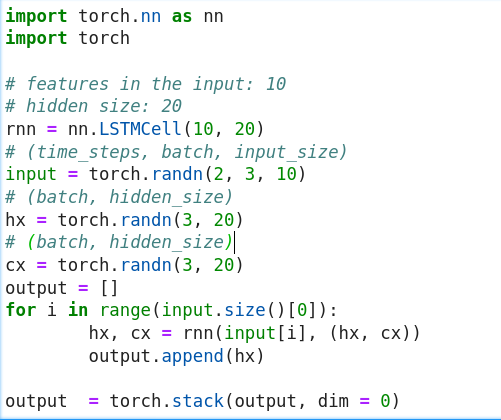# LSTMCell Example

Hi, I think that there is an error in the example presented for a LSTMCell:
https://pytorch.org/docs/stable/generated/torch.nn.LSTMCell.html:

The loop iterates over 6 steps, but the input has only 3 steps. I also think that there is an error with the shape of the initial hidden and cell states. Here is my suggestion:

rnn = nn.LSTMCell(10, 20)
input = torch.randn(3, 10)
hx = torch.randn(1, 20)
cx = torch.randn(1, 20)
output = []
for i in range(input.size()):
hx, cx = rnn(input[i:i+1], (hx, cx))
output.append(hx)

Please let me know if I misunderstood somethingThanks for pointing out this issue. Could you create an issue on GitHub, so that we can track and fix it?
Based on the current code snippet I assume the example should use `6` time steps, so `input` would have to be initialized as e.g. `torch.randn(6, 3, 10)`.

Ok, I did it ( LSTM Cell example #51801). I suggested the example of the GRUCell adapted to the gates of an LSTM: